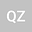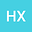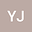Ground state solutions of Poho\v{z}aev type for Kirchhoff type problems with general convolution nonlinearity and variable potential
•••Hai Xie
Guilin University of Technology
Author ProfileYirong Jiang
Guilin University of Technology
Author Profile## Abstract

This paper is devoted to dealing with the following nonlinear Kirchhoff type problem with general convolution nonlinearity and variable potential: $$\left\{\begin{array}{ll} -({a + b\int_{{\R^3}} | \nabla u{|^2}dx})\Delta u + V(x)u =(I_{\alpha}\ast F(u))f(u),\quad \text{in}\ \ \R^3,\\ u \in H^{1} (\R^{3}), \end{array}\right.$$ where $a>0$, $b\geq0$ are constants, $V\in C^1(\R^3,[0,+\infty))$, $f\in C(\R,\R)$, $F(t)=\int_{0}^{t}f(s)ds$, $I_{\alpha}:\R^{3}\rightarrow \R$ is the Riesz potential, $\alpha\in(0,3)$. By applying some new analytical tricks introduced by [X.H. Tang, S.T. Chen, Adv. Nonlinear Anal. 9 (2020) 413-437], the existence results of ground state solutions of Poho\v{z}aev type for the above Kirchhoff type problem are obtained under some mild assumptions on $V$ and the general “Berestycki-Lions assumptions” on the nonlinearity $f$. Our results generalize and improve the ones in [P. Chen, X.C. Liu, J. Math. Anal. Appl. 473 (2019) 587-608.] and other related results in the literature.
21 Feb 2022Submitted to Mathematical Methods in the Applied Sciences
22 Feb 2022Assigned to Editor
22 Feb 2022Submission Checks Completed
08 Mar 2022Reviewer(s) Assigned
28 Jun 2022Review(s) Completed, Editorial Evaluation Pending
29 Jun 2022Editorial Decision: Accept